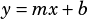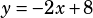Which of the following equations, together with 2x + y= 8, results in a system of equations with exactly one solution?

A) -2x – y= -8
B) x+2y=0
C) 2x+y=0
D) 2x+y=14
E) 4x+2y= 16

These are all linear equations, so they’ll either have one solution (if the lines intersect), no solutions (if the lines are parallel), or infinite solutions (if the lines are actually just the same line).

Since you want one solution, you want lines that will intersect, which means you want lines that don’t have the same slopes. Put the original equation into slope-intercept () form:I won’t put all the answer choices into that form (you can do that yourself) but I will point out that the correct answer choice, B, looks like this in slope-intercept form:If two lines have slopes ofand, then they aren’t parallel, so they intersect.